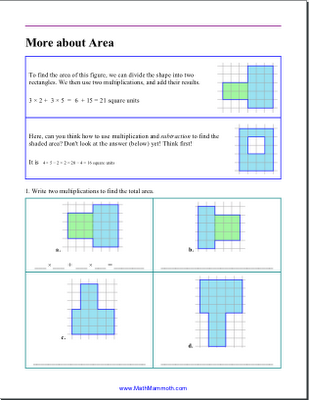Here's a little sample of what is keeping me so busy right now - third grade math, aligning things to the Common Core standards.

This is a complete lesson (4 pages) about area for grade 3 math. It's not the initial lesson - it assumes the student already knows how to find the area of a rectangle. (Sorry - there is no answer key at this time; it hasn't been made yet!)The lesson (worksheets) is specifically about these two standards:

3.MD

7.c. Use tiling to show in a concrete case that the area of a rectangle with whole-number side lengths a and b + c is the sum of a × b and a × c. Use area models to represent the distributive property in mathematical reasoning.

d. Recognize area as additive. Find areas of rectilinear figures by decomposing them into non-overlapping rectangles and adding the areas of the non-overlapping parts, applying this technique to solve real world problems.Parshall Family said…
Maria, I have a rising seventh grader who is ready for pre-algebra. Have you looked at the often-recommended texts like Lial's Prealgebra to see how they measure up to the common core standards? I would like whatever textbook we choose to meet the CCS even though I don't necessarily agree with the philosophy behind them.Maria Miller said…
No, I haven't looked at the common textbooks to compare them to the standards. However, typical pre-algebra books should approximately cover the main areas of the standards for 7th grade. Probability/statistics is the main area where pre-algebra books may fail.

Here is an overview of Common Core standards for math for 7th grade.

Ratios and Proportional Relationships Mathematical Practices
* Analyze proportional relationships and use them to solve real-world and mathematical problems.

The Number System
* Apply and extend previous understandings of operations with fractions to add, subtract, multiply, and divide rational numbers.
(This means integer arithmetic.)

Expressions and Equations
* Use properties of operations to generate equivalent expressions.
* Solve real-life and mathematical problems using numerical and algebraic expressions and equations.

Geometry
* Draw, construct and describe geometrical figures and describe the relationships between them.
* Solve real-life and mathematical problems involving angle measure, area, surface area, and volume.

Statistics and Probability
* Use random sampling to draw inferences about a population.
* Draw informal comparative inferences about two populations.
* Investigate chance processes and develop, use, and evaluate probability models.Mensuration is a very important topic of mathematics as it is very much related to our daily life like area and volume of different solid figures or area of plot,length and breadth of different object.It is interlinked with many real life problems.